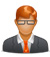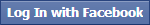## Question## How to calculate GATE 2014 Score ?

Hello sir,
I am a GATE 2014 aspirant; I just want to know h
ow I can calculate my GATE score, any specific formula is us
ed to calculate score and What GATE score is required to get
Thanks

Asked by 2427 days ago- by RoyabhiGATE exam score is used to get admission in M.Tech programmes and other master degrees in engineering offered by IITs and IISc of the country. Here is the formula to calculate GATE score:

The GATE percentile is calculated as follows:

P = ( (N- your rank)/ N) x 100

Where P is percentile and N is total number of candidates appeared for exam.

(N- Your rank) indicates the number of candidates who have scored less marks than you.

For example, if total number of candidates are 100 and 90 of them have less marks than you P = (09/100)X100 = 0.9×100 = 90 percentile.

For another example: A guy form Mechanical Engineering have All India Rank (AIR) 99 and total number of student of students appeared for GATE in Mechanical Engineering are 59338 in 2010. Then his percentile would be calculated as

P = ((59338-99)/ 59338) X 100

= (59239/59338) X 100

= (0.9983) X 100

= 99.83

The candidate has 99.83 percentile.

Candidates appear in GATE exam to get admission in IITs and IISc of the country. But here comes the big question how much percentile is enough to get admission. This is really competitive exam and even 99+ percentile will make you confuse about the admission. IITs take their own written test and interview to enrol students.

There are nearly 50-60 seats in every IITs in each department. And considering only old IITs take admission for M.Tech, there will be 7X50= 350 seats for M.Tech in IITs for almost every department.

Response by Expert , 2427 days ago

### Featured Questions

Top 10 MBA colleges & admission procedure
Asked 2574 days ago- by Prasanth Kumar Mannem
MAT paper pattern & qualifying marks
Asked 2574 days ago- by Rahul Joshi
Top 10 engineering colleges in world
Asked 2583 days ago- by Rajendra Rabha
Eligibility requirement to appear GMAT
Asked 2583 days ago- by Prasanth Kumar Mannem
MBA from India and MBA from Abroad
Asked 2574 days ago- by Gayathri.k
Confuse between MBA & MCA
Asked 2587 days ago- by Gayathri.k
Top MBA Institutes of India and their admission process
Asked 2589 days ago- by Nilesh Ahire
Asked 2589 days ago- by KALYAN DAS

### Sign Up ORCountry CodeNumberNot readable?